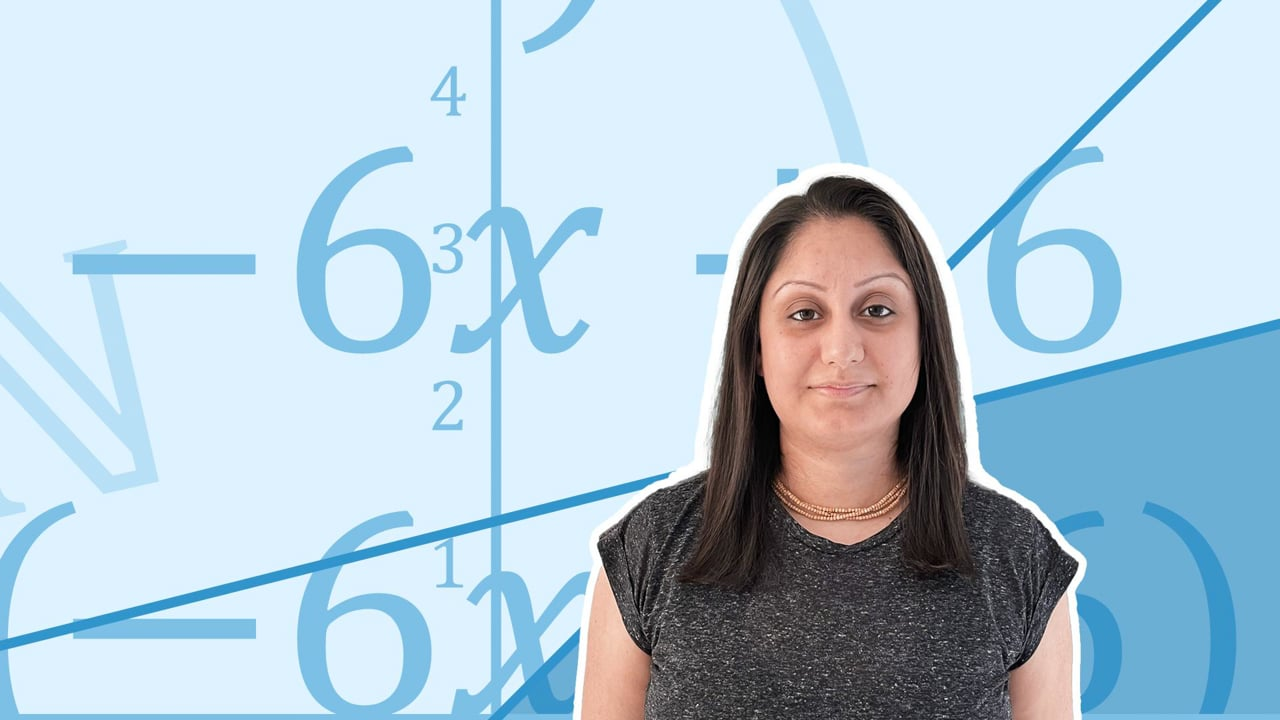Chapter overviewMaths

Exam board

AQA

Number

Algebra

Graphs

Ratio proportion and rates of change

Shapes and area

Angles and geometry

Trigonometry

Probability

Statistics

Maths

# Single brackets: Expanding and factorising0%

Summary

# Single brackets: Expanding and factorising

## In a nutshell

A number or term can be multiplied by an expression in brackets. e.g. $2x(3x+2y)$. Here, the term on the outside of the bracket should be multiplied by each term in the expression that is inside the brackets. This is called multiplying out single brackets. Some expressions can be put back into single brackets, by taking out a common factor from the expression. This is called factorising single brackets.

Note: Be careful with negative signs inside the bracket, or with a negative term in front of the bracket.

## Multiplying out single brackets

To multiply out brackets, multiply the number on the outside with each term on the inside of the bracket. You can use a multiplication grid to help.

##### Example 1

Multiply out $2(x+3)$.

Use a multiplication grid to help.

 ​$\times$​​ ​$x$​​ ​ $+3$​​ ​$2$​​ ​$2x$​​ ​$6$​​

##### Examples
 ​$3(y+1)$​​ ​$= 3y+3$​​ ​$2x (x-5)$​​ ​$=2x^2 - 10x$​​ ​$3a(2b+5t)$​​ ​$=6ab+15at$​​ ​$-5x(3x-4y)$​​ ​$=-15x^2+20xy$​​ ​$3b(2c^2+7d-3)$​​ ​$=6bc^2+21bd-9b$​​

## Factorise single brackets

To factorise single brackets, think about what is common in all the terms in the expression. This is the common factor. Keep the common factor on the outside of the bracket, and find the expression on the inside of the bracket.

##### Example 2

Factorise $3x+12$.

$3x$ and $12$ both have a common factor of $3$. So take the number $3$ on the outside of the bracket. Then you need $x$, to multiply with $3$ to get $3x$, and $+4$ to multiply by $3$ to get $+12$.

$3x+12 = \underline{3(x+4)}$

You can use a multiplication grid to help.

 ​$\times$​​ ​$x$​​ ​$+4$​​ ​$3$​​ ​$3x$​​ ​$+12$​​

## Want to find out more? Check out these other lessons!

Single brackets: Expanding and factorising

FAQs

• Question: What is a common factor when factorising single brackets?

Answer: A common factor is a number or term that divides exactly into 2 or more terms in an expression. E.g. For the expression 3x+12, 3 divides into 3x exactly, and 12 exactly, so 3 is the common factor.

• Question: How do you factorise single brackets?

Answer: To factorise single brackets, think about what is common in all the terms in the expression. This is the common factor. Keep the common factor on the outside of the bracket, and find the expression on the inside of the bracket.

• Question: How do you multiply out single brackets?

Answer: To multiply out single brackets, make sure to multiply the term on the outside of the bracket with each term on the inside of the bracket.

Theory

Exercises